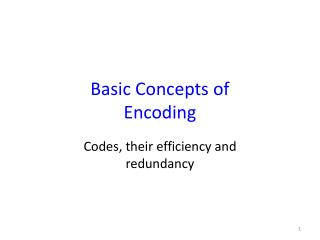DownloadDownload PresentationBasic Concepts of Encoding

# Basic Concepts of Encoding

Download Presentation## Basic Concepts of Encoding

- - - - - - - - - - - - - - - - - - - - - - - - - - - E N D - - - - - - - - - - - - - - - - - - - - - - - - - - -
##### Presentation Transcript

1. Basic Concepts of Encoding Codes, their efficiency and redundancy

2. Cost function • How we can measure the efficiency of encoding? • The ability to correct the errors is a great “plus”, but we have realized that a code, which is able to correct the errors, is redundant. Where there is an equilibrium point between redundancy and efficiency? • Is it possible to compress the input information using encoding? • To ensure more efficient encoding, we should introduce its cost function. In this terms, the efficient encoding will mean minimization of this function.

3. Cost function • Let be a set of messages (words) that can be transmitted through the communication system and be their “prescribed” probabilities, and be their durations. • The duration of a message may be considered as its cost. Thus, the simples cost function is the average (statistical) cost per message is

4. Cost function • Suppose (without loss of generality) that all symbols in all messages have identical cost. Thus, the average cost per message becomes proportional to the average number of symbols per message, that is, the average cost per message = the average length of messages:

5. Cost function and efficiency • An increase in transmission (representation) efficiency can be obtained by such a method of encoding that the statistical distribution of the symbols used for it reduce the average word length . • The efficiency of the encoding procedure can be defined, if, and only if, we know the lowest possible bound of .

6. Efficiency • It was shown that the lower bound for the average word length is the ratio of the entropy of the original message ensemble to log D, where D is the number of symbols in the encoding alphabet: H(X)/log D • log D is the maximum possible information per symbol.

7. Efficiency • The efficiency of an encoding procedure is the ratio of the average information per symbol of encoded language to the maximum possible average information per symbol:

8. Redundancy • The redundancy of the code is defined as follows:

9. Efficiency and Redundancy • Example 1. Suppose, we transmit four messages that are the letters of the encoded alphabet:

10. Efficiency and Redundancy • Example 2. Let us encode a set of the same four messages using a binary alphabet: . Let and , k=1,2,3,4, be the numbers of 0’s and 1’s in the encoding binary vector (word), nk be the length of the encoding word.

11. Efficiency and Redundancy • The average cost (the average length) of message is and • Hence, the direct uniform encoding for this certain input set of messages does not improve the efficiency

12. Efficiency and Redundancy • Example 3. Let us use a non-uniform code for the same example • A simple logic leads to the following idea: encoding a frequent message by a shorter encoding vector (word) and a less frequent message by a longer encoding vector (word)

13. Efficiency and Redundancy • Let us use the following encoding vectors: • Then

14. Efficiency and Redundancy • The last example shows that it is possible to design a non-uniform code with the 100% efficiency. • Moreover, this code makes it possible to compress the input information: the average length of the encoding vector in the uniform code was 2, while in the non-uniform code, which we have just considered, it was 1.75

15. Decipherability and Irreducibility • The code has the following important property: any message composed from these encoding vectors independently of their amount and order always can be unambiguously decoded. This property of code is referred to as unique decipherability.

16. Decipherability and Irreducibility • A sufficient condition for unique decipherability of a non-uniform code is that no encoding vectors (words) can be obtained from each other by the addition of more letters to the end. This property is referred to as the prefix property or irreducibility. This means that there is no encoding vector, which is a prefix of the different encoding vector.

17. Decipherability and Irreducibility • The set of irreducible codes is a subset of the set of uniquely decipherable codes. If a code is irreducible, then it is definitely uniquely decipherable, but the opposite is not true. • For example, a code (1) (10) is uniquely decipherable, but not irreducible: (1) is the prefix for (10).